﻿ 一类(2 + 1)维KdV方程的精确解及动力学性态

# 一类(2 + 1)维KdV方程的精确解及动力学性态Dynamical Behaviors and Solutions of a Class of (2 + 1)-Dimensional KdV Equations

Abstract: In this thesis, new exact solutions to generalized two dimensional KdV equation with variable coefficients were constructed with exp-function method and homogeneous balance method, and graphic representation of the solutions was obtained and properties of the solutions were analyzed with Mathematica software. With new exact solutions to KdV equations obtained, the influence of each parameter on waves was observed and the degree to which each factor influences the propagation state of water waves was analyzed, which provided certain reference to problems regarding the propagation of water waves under shallow-water background.

1. 引言

${\left[{u}_{t}+{\text{6}uu}_{x}+{u}_{xxx}\right]}_{x}+a\left(t\right){u}_{x}+b\left(t\right){u}_{yy}+{F}_{xx}\left(x,y,t\right)=0.$

${\left[{u}_{t}+\text{6}u{u}_{x}+{u}_{xxx}\right]}_{x}+2{u}_{yy}=0.$ (1)

2. 方程求解

2.1. 方法概述

$P\left({u}_{t},{u}_{x},{u}_{tx},{u}_{xx},{u}_{xxx},\cdots \right)=0.$ (2)

1) 假设非线性偏微分方程(2)的解为 $u\left(x,t,y\right)=U\left(\eta \right)$，其中 $\eta =kx+t+y$ 将，则偏微分方程(2)转化为非线性常微分方程：

$Q\left({U}^{\prime },{U}^{″},{U}^{‴},\cdots \right)=\text{0}\text{.}$

2) 假设方程(2)具有以下形式的解

$U\left(\eta \right)=\frac{\underset{n=-d}{\overset{c}{\sum }}{a}_{n}\mathrm{exp}\left(n\eta \right)}{\underset{m=-q}{\overset{p}{\sum }}{b}_{m}\mathrm{exp}\left(m\eta \right)},$

3) 通过平衡指数函数的幂次得到关于 ${a}_{n}$${b}_{m}$ 超定代数方程  来确定，求解代数方程组即可得到(2)精确解。

2.2. 方程(1)的精确解

$6{\left(k{U}^{\prime }\right)}^{2}+\left(k+2\right){U}^{″}+6{k}^{2}U{U}^{″}+{k}^{4}{U}^{\left(4\right)}=0.$ (3)

$U\left(\eta \right)=\frac{\underset{n=-d}{\overset{c}{\sum }}{a}_{n}\mathrm{exp}\left(n\eta \right)}{\underset{m=-q}{\overset{p}{\sum }}{b}_{m}\mathrm{exp}\left(m\eta \right)}.$ (4)

$\text{2}c\eta +3d\eta =c\eta +4d\eta$

$-3p\eta -2q\eta =-4p\eta -q\eta$

$c=p=1,\text{}d=q=1$，代入方程(4)得到方程：

$U\left(\eta \right)=\frac{{a}_{-1}+{e}^{\eta }\left({a}_{0}+{e}^{\eta }{a}_{1}\right)}{{b}_{-1}+{e}^{\eta }\left({b}_{0}+{e}^{\eta }{b}_{\text{1}}\right)}.$ (5)

2.3. 图像分析

1)

${U}_{\text{1}}=-\frac{{k}^{4}+k+2}{6{k}^{2}}+\frac{4{k}^{2}{b}_{-1}{b}_{0}{\text{e}}^{kx+t+y}}{{\left(2{b}_{-1}+{b}_{0}{\text{e}}^{kx+t+y}\right)}^{2}},$

${U}_{1}=-\frac{\text{5}}{\text{6}}+\frac{\text{96}{e}^{2x+\text{t}+y}}{{\left(6+2{e}^{2x+t+y}\right)}^{2}}.$

$x\in \left(-10,10\right),\text{}y\in \left(-10,10\right)$，画出以下图像。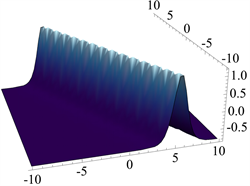Figure 1. When t = 0Figure 2. When t = 10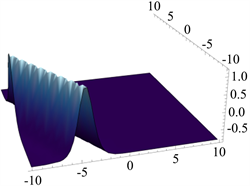Figure 3. When t = 20

2)

${U}_{2}=-\frac{\left({k}^{4}+k+2\right)\left(144{k}^{4}{\text{e}}^{2kx+2t+2y}+24{k}^{2}\left(5{k}^{4}-k-2\right){b}_{0}{\text{e}}^{kx+t+y}+{\left({k}^{4}+k+2\right)}^{2}{b}_{0}^{2}\right)}{6{\left(-12{k}^{3}{\text{e}}^{kx+t+y}+k\left({k}^{4}+k+2\right){b}_{0}\right)}^{2}},$

${U}_{\text{2}}=-\frac{\text{10}\left(\text{400}+\text{7296}{e}^{t+2x+y}+2304{e}^{2\left(t+2x+y\right)}\right)}{\text{3}{\left(\text{40}-\text{96}{e}^{t+2x+y}\right)}^{2}}.$

$x\in \left(-10,10\right),\text{}y\in \left(-50,50\right)$ 时，画出图像。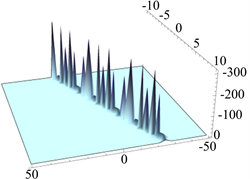Figure 4. When t = 5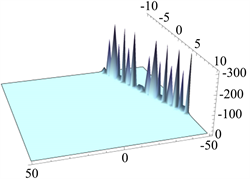Figure 5. When t = 40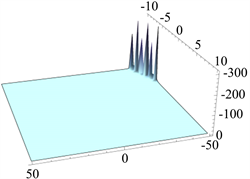Figure 6. When t = 60

3)

${U}_{3}=-\frac{\left({k}^{4}+k+2\right)\left(144{k}^{4}+{b}_{0}{\text{e}}^{kx+t+y}\left(24{k}^{2}\left(5{k}^{4}-k-2\right)+{\text{e}}^{kx+t+y}{\left({k}^{4}+k+2\right)}^{2}{b}_{0}\right)\right)}{6{\left(-12{k}^{3}+k\left({k}^{4}+k+2\right){b}_{0}{\text{e}}^{kx+t+y}\right)}^{2}},$

$k=2$${b}_{0}=1$，则精确解简化为：

${U}_{\text{3}}=-\frac{\text{10}\left(\text{2304}+{\text{e}}^{t+2x+y}\left(7296+400{\text{e}}^{t+2x+y}\right)\right)}{\text{3}{\left(-\text{96}+{\text{40e}}^{t+2x+y}\right)}^{2}}.$

$x\in \left(-10,10\right)$$y\in \left(-50,50\right)$ 时，画出图像：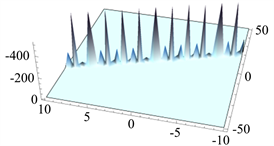Figure 7. When t = 1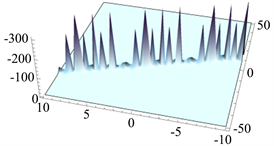Figure 8. When t = 10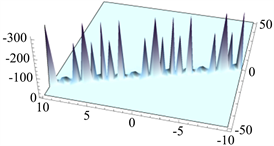Figure 9. When t = 20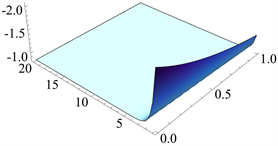Figure 10. When t = 1

3. 结论

 余招贤. 分离变量法的适用条件[J]. 科教文汇, 2017(33): 36-37.

 Wang, M.L., Zhou, Y.B. and Li, Z.B. (1999) Application of a Homogeneous Balance Method to EXACT solution of Nonlinear Equations in Mathematical Physics. Physics Letters A, 216, 67-75.
https://doi.org/10.1016/0375-9601(96)00283-6

 郭玉翠. 非线性偏微分方程引论[M]. 北京: 清华大学出版社, 2008: 211-215, 105-122, 216-221, 243.

 张佳梅, 等. 一个广义变系数KdV方程新的精确解[J]. 应用数学进展, 2013, 2(1): 42-47. http://dx.doi.org/10.12677/AAM.2013.21006

 He, J.H. and Wu, X.H. (2006) Exp-Function Method for Nonlinear Wave Equations. Chaos, Solitons & Fractals, 30, 700-708.
https://doi.org/10.1016/j.chaos.2006.03.020

 Wu, X.H. and He, J.H. (2007) Solitary Solutions Periodic Solutions and Compacton-Like Solutions Using the Exp- Function Method. Computers & Mathematics with Applications, 54, 966-986.
https://doi.org/10.1016/j.camwa.2006.12.041

 胡恒春, 王利金, 刘磊. KdV-Burgers-Kuramoto方程另一类指数函数求法及新的精确解[J]. 上海理工大学学报, 2013, 35(2): 131-134.

 向欢. 一类非线性超定方程组数值解法的研究[J]. 数学理论与应用, 2017, 37(3): 17-25.

 李国, 蒋彦, 杨东升, 梁宏军. Mathematica软件函数图形绘制及特性分析中的应用[J]. 现代电子技术, 2006, 29(22): 59-60+66.

Top